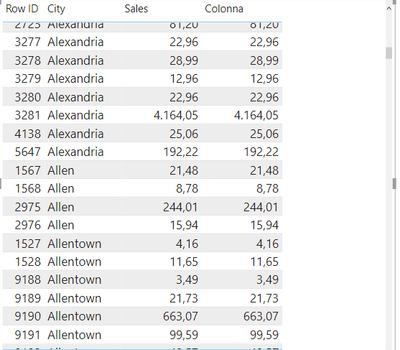cancel
Showing results for
Did you mean:Helper III

## dax formula for column

Hi,

I need to make a new COLUMN that shows the aggregated value of sales based on a city ( as a result I need to see the total number of sales fot each city for example for city Allen I need to see 290.21)

How can I do?

Thanks1 ACCEPTED SOLUTIONSuper User

new column =

sumx(filter(Table, Table[City] = earlier(Table[City]) ), [Sales])

or

new measure=

sumx(filter(allselected(Table), Table[City] = max(Table[City]) ), [Sales])Super User

new column =

sumx(filter(Table, Table[City] = earlier(Table[City]) ), [Sales])

or

new measure=

sumx(filter(allselected(Table), Table[City] = max(Table[City]) ), [Sales])